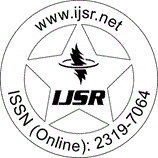International Journal of Science and Research (IJSR)
Call for Papers | Fully Refereed | Open Access | Double Blind Peer Reviewed

Research Paper | Electronics Engineering | India | Volume 2 Issue 7, July 2013

# Quaternary Arithmetic Logic Unit Design Using VHDL

Prashant Y. Shende | R. V. Kshirsagar

Abstract: The arithmetic logic unit (ALU) is the core of a CPU in a computer; the adder cell is the elementary unit of an ALU. The constraints the adder has to satisfy are area, power and speed requirements. The delay in an adder is dominated by the carry chain. Arithmetic operations such as addition, subtraction and multiplication still suffer from known problems including limited number of bits, propagation time delay, and circuit complexity. Carry free arithmetic operations can be achieved using a higher radix number system such as quaternary Signed Digit (QSD). We proposed fast arithmetic logical unit based on quaternary signed digit number system where the carry propagation chain are eliminated, hence it reduce the propagation time in comparison with radix 2 system, each digit can be represented by a number from -3 to -3. In any n digit QSD number, each digit can be represented by a number from the digit set [-3, -2, -1, 0, 1, 2, 3]. Operations on a large number of digits can be implemented with constant delay and less complexity.

Keywords: quaternary sign digit, fast computation, multiplier, quaternary logic, Carry/borrow free

Edition: Volume 2 Issue 7, July 2013,

Pages: 268 - 271